## research papersJOURNAL OFSYNCHROTRONRADIATION
ISSN: 1600-5775
Volume 18| Part 3| May 2011| Pages 522-526

## X-ray collimation by crystals with precise parabolic holes based on diffractive–refractive optics

aInstitute of Physics, Academy of Sciences of the Czech Republic, v.v.i., Na Slovance 2, CZ-18221 Praha 8, Czech Republic, bSwiss Light Source, Paul Scherrer Institut, CH-5235 Villigen, Switzerland, and cDepartment of Condensed Matter Physics, Faculty of Science, Masaryk University, Kotlářská 2, CZ-61137 Brno, Czech Republic
*Correspondence e-mail: peter.oberta@psi.ch

(Received 21 May 2010; accepted 9 March 2011; online 1 April 2011)

Two crystals with precise parabolic holes were used to demonstrate sagittal beam collimation by means of a diffractive–refractive double-crystal monochromator. A new approach is introduced and beam collimation is demonstrated. Two Si(333) crystals with an asymmetry angle of α = 15° were prepared and arranged in a dispersive position (+,−,−,+). Based on theoretical calculations, this double-crystal set-up should provide tunable beam collimation within an energy range of 6.3–18.8 keV (ΘB = 71–18.4°). An experiment study was performed on BM05 at ESRF. Using 8.97 keV energy, the beam profile at various distances was measured. The experimental results are in good agreement with theoretical predictions. Owing to insufficient harmonic suppression, the collimated (333) beam was overlapped by horizontally diverging (444) and (555) beams.

### 1. Introduction

Beam shaping based on diffractive–refractive optics has been previously studied and demonstrated by our group at the Institute of Physics of the Academy of Sciences of the Czech Republic. Both Bragg (Hrdý, 1998) and Laue (Hrdý et al., 2006) diffraction approaches have been demonstrated. Based on theoretical calculations (Hrdý & Oberta, 2008) and ray-tracing simulations (Artemiev et al., 2004), various applications for diffractive–refractive optics were proposed and tested at synchrotron facilities. Recently, the smallest focal spot size by diffractive–refractive optics (Oberta et al., 2010) was achieved and a novel method of higher harmonics separation in space was proposed at the Swiss Light Source (SLS; Hrdý et al., 2011). In addition to beam focusing, another application of diffractive–refractive optics is beam collimation. In this paper a new approach is introduced and beam collimation is demonstrated using a dispersive arrangement of two crystals with precise parabolic holes.

There are a number of methods used to collimate X-rays. Mirrors or asymmetrically cut crystals can be used (Mori & Sasaki, 1995; Renninger, 1961), but these approaches change the direction of beam propagation and additional optics are needed to correct this deviation. Our method is based on two asymmetrically cut crystals into which we machined holes with a precise parabolic profile: two channel-cut crystals. The dispersive arrangement has the advantage, compared with other methods, that it conserves beam direction: the entrance and exit beam positions are fixed. The same advantage holds for refractive lenses (Snigirev et al., 1996); however, they can only be used for hard X-ray radiation. Another advantage of the diffractive–refractive method is lower flux loss compared with other collimation methods. The Si(333) reflection loses just about 10% of flux after diffraction.

### 2. Theoretical description

Imagine a crystal into which a hole is drilled. This hole is further machined to reach an ideal parabolic shape. The crystal is cut in such a way that the crystallographic planes of the (111) orientation form an angle α with the crystal surface (asymmetric crystal), Fig. 1. If one takes a point source, located at a distance S from the crystal, then the diffracted radiation from the parabolic surface profile of the drilled hole in the crystal will be sagittally focused to a point at a distance f from the crystal. The formula describing the parabolic profile can be expressed as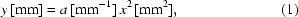where a is the parabola parameter. Then the relationship between S, f and a can be expressed as (Hrdý & Oberta, 2008; Snigirev et al., 1996)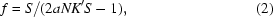where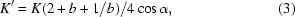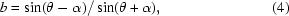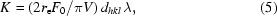where for siliconHere re is the classical electron radius, F0 is the structure factor, V is the unit-cell volume, λ is the wavelength, dhkl is the atomic plane spacing, α is the asymmetry angle (α > 0 for the grazing-incidence case), θ is the Bragg angle, N is the number of diffraction events and b is the asymmetry factor. A plot of formula (2)for dhkl = (111) shows the dependence of the focusing distance over energy or Bragg angle (Oberta et al., 2010). By using only one crystal with a parabolic shaped hole, Fig. 1, the number of diffracting events is two, N = 2. As shown by Hrdý & Siddons (1999), such a crystal arrangement cannot achieve a sharp focal spot, because of the combined vertical and horizontal spread of the beam. To cancel the vertical and horizontal beam spreads, a Bartels crystal arrangement must be used. For this case the number of diffracting events is four, N = 4 (Fig. 2). A single crystal with two diffracting events, N = 2, is a non-dispersive system, but the two-crystal system used in the experiment represents a dispersive system, with N = 4 diffracting events (Fig. 2).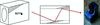Figure 1 A silicon Si(111) asymmetrically cut crystal with a parabolic-shaped drilled hole and double-bounce beam path inside the crystal.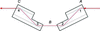Figure 2 The two-crystal dispersive system with four diffraction events. The four diffraction events are in reality separated in space (1–4). The theory simplifies them into one point in space.

The focusing condition is sensitive to dispersion and thus depends on the reflection. Fig. 3shows the focusing distance over a Bragg angle of a dispersive arrangement of two crystals with a parabolic-shaped drilled hole for the Si(333) crystal orientation. The crystal–source distance was S = 33613 mm as used at BM05 at ESRF where the experiment was performed. The parabola parameter of our crystals is a = 0.15 mm−1. As can be seen from Fig. 3, for certain Bragg angles (energies) the focusing distance is several hundred meters from the crystal or becomes infinite (inclined dashed area). Under these conditions the crystal arrangement is a collimator. The collimation is therefore independent of the divergence of the impinging beam. This is the working principle of the diffractive–refractive X-ray collimator.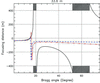Figure 3 Focusing distance as a function of Bragg angle for diffractions Si(333) (black full line), Si(444) (red dash-dotted line) and Si(555) (blue dashed line). The gray inclined dashed areas represent the working range of the collimator for a crystal–source distance of 33.6 m.

Fig. 3plots the calculated focal distance for three different harmonics. The black full line represents the Si(333) focusing versus Bragg angle dependence. We can see higher harmonics, plotted as red dash-dotted [Si(444)] and blue dashed [Si(555)] lines. A negative focusing distance means a divergence of the beam with a focal point placed before the crystals. A positive focusing distance represents focusing with the focal point placed after the crystals. If a focusing distance of an optical set-up, like the proposed crystal arrangement, is from several hundred meters up to kilometers; such a set-up can be described as collimating. For the case of the diffractive–refractive X-ray collimator this value was set to ±500 m. The focusing distance is very sensitive to the crystal–source distance S (Fig. 4). By changing S and moving the crystals over a range of 10 m, we can achieve a collimation effect over an angular range of ΘB = 71–18.4°, that corresponds to an energy range of 6.3–18.8 keV. Because the proposed crystal collimator is based on the diffractive–refractive effect, which is much stronger for longer wavelengths, the working range of the collimator lies in the soft X-ray region. In Fig. 4we find two collimating angular ranges (inclined dashed area). The range for larger ΘB (smaller energies) is broader than that for smaller ΘB (larger energies). Both ranges are approaching each other with increasing distance S.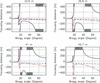Figure 4 Focusing distance versus Bragg angle for four different crystal–source distances. With increasing crystal–source distance the two working ranges are approaching each other.

Table 1includes the tabulated angular and energy ranges for eight different crystal–source distances, along with the focal distances of some harmonics. Table 2also includes the tabulated broadening of the beam compared with an ideal collimation as a percentage of the impinging beam dimensions. The additional broadening of the Si(333) diffraction for a focal distance of 500 m is within ∼0.18% of ideal, which justifies our approximation approach. In the case of the higher harmonics the share to the beam broadening is higher, but still in the range between 5.8% and 14%.

 Table 1Angular and energy ranges for eight different crystal–source distances, S, along with the focal distances of some harmonics
S (m) Collimation range (°) Collimation range (keV) f333 (m) f444 (m) f555 (m)
32.8 18–19/53.5–71 19.2–18.23/7.38–6.27 ±500 −69.29 −49.52
34.8 18.4–19.4/47.5–62 18.81–17.86/8.05–6.72 ±500 −77.26 −53.73
36.8 19–20/43–56 18.23–17.36/8.7–7.15 ±500 −87.86 −58.65
38.8 19–21/38–51 18.23–16.57/9.64–7.63 ±500 −100 −63
40.8 20–22/33.5–46.5 17.36–15.85/10.75–8.17 ±500 −97 −65
42.8 20–43 17.36–8.7 ±500 −93 −70
44.8 21–39.5 16.57–9.33 ±500 −111 −72
46.7 22–37 15.85–9.86 ±500 −118 −76
 Table 2Broadening of the beam compared with an ideal collimation as a percentage of the impinging beam dimensions
S (m) Si(333) Si(444) Si(555)
32.8 0.18 9.8 14.0
34.8 0.18 9.0 13.0
36.8 0.18 7.8 11.8
38.8 0.18 7.0 11.0
40.8 0.18 7.2 10.6
42.8 0.18 7.4 10.0
44.8 0.18 6.2 9.6
46.7 0.18 5.8 9.2

As one can see from Fig. 4and Table 2, harmonic contamination will project itself as an additional beam broadening and will decrease the multiwavelength collimation. One can overcome this problem by using a special crystal geometry to separate the higher harmonics in space without deforming the reflectivity curve, as carried out by traditional harmonics separation methods (Hrdý et al., 2011), or by introducing a slit system between the two crystals.

### 3. Experimental results

The experiment was performed on a vertical diffractometer at the BM05 beamline at ESRF. We set two crystals with precise machined parabolic holes into a dispersive position and set the beamline monochromator to 8.97 keV.

First we set only one crystal into the diffraction position (ΘBSi(333) = 41.4°) and detected the diffraction. Then we removed the crystal and set the second crystal. After adjusting the second crystal we set both crystals in-line. At different distances we recorded diffraction images on X-ray films and on a digital camera.

#### 3.1. Single-crystal arrangement

In a set-up with only one crystal diffracting we detected higher harmonics. As can be seen in Fig. 5, there are two parabolic-shaped diffraction spots on the X-ray film. The full parabolic diffraction spot corresponds to the Si(333) diffraction, which copies the shape of the parabolic groove. Based on the distance of 7 m of the X-ray film and the angular separation of 0.7° of the two diffractions one can calculate the corresponding energy of the other diffraction. Based on this approach we found out that the partial parabolic diffraction corresponds to the Si(444) diffraction energy.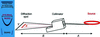Figure 5 Diffractions after one crystal at a distance of 7 m. The horizontal line spot is the primary beam propagating through the crystal, the full parabolic spot is the Si(333) diffraction, and the partial parabolic double-spot is the Si(444) diffraction. Owing to the diffractive–refractive effect the harmonics are separated in space.

After diffraction from one crystal the higher harmonics are separated in space (Hrdý et al., 2011), and after two crystals the higher harmonics are assembled again in-line and overlap each other. Therefore, higher harmonics contamination could be solved by introducing a slit system between the two crystals. The schematic in Fig. 5shows the layout of the experiment. Here A is the crystal–source distance (33600 mm), B is the crystal–X-ray film distance (7000 mm), X is the Si(333) diffraction spot size [width of the Si(333) parabolic diffraction, 9.05 mm] and Y is the width of the primary beam, 10.9 mm. These dimensions are related as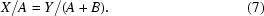From this simple relationship we can calculate the width of the Si(333) collimated diffraction spot if the collimation is ideal. For the ideal collimation the Si(333) diffraction spot is 9.02 mm. The error between experiment (9.05 mm) and calculation (9.02 mm) is just 0.3%.

#### 3.2. Double-crystal arrangement

By assembling the two crystals in-line, higher harmonics, which were spatially separated by the first crystal, are assembled again together by the second crystal. This way the spot after the two crystals is contaminated by higher harmonics, which are not collimated and degrade the apparent collimation effect. Also the parabolic-shaped diffraction after one crystal is canceled by the second crystal. As a result we restore a line beam (Figs. 6and 7). By rocking one of the crystals (Fig. 6), higher harmonics are visible. The diffraction in Fig. 6has a FWHM of 10.4 arcsec, which corresponds to the Si(444) diffraction at 8.97 keV with a theoretical FWHM of 10.6 arcsec. The second diffraction spot, visible in frames 3–8, is the diffracted Si(333). Fig. 7shows an X-ray film image of diffraction after two crystals at a distance of 7 m after the crystals. The false colors represent the different energies. The red color denotes the diffraction spot of the Si(333) diffraction, green the Si(444) diffraction, and light blue for the Si(555) diffraction. The dimensions of the diffraction footprint in Fig. 7correspond to the theoretical spread of the higher harmonics calculated from Fig. 3. The theoretical broadening of the beam after a slit size of 5 mm should be 5.16, 5. 48 and 5.67 mm for the Si(333), Si(444) and Si(555) diffraction, respectively. The beam dimensions obtained by experiment were 5.12, 5.44 and 5.70 mm for the Si(333), Si(444) and Si(555) diffraction, respectively. The theoretical description (Hrdý & Oberta, 2008; Snigirev et al., 1996) supposes that all the diffraction events occur in one point in space; this way a clear crystal–source distance is set. In reality there are four different points of diffraction in the two crystals; the diffracted beam bounces twice from each crystal. Contrary to theory, we do not have one spatial point for all four diffracting events and one crystal–source distance, but four points and four different crystal–source distances. This situation can be simplified to one of the three different points in Fig. 2. We can either choose the first diffraction event at the first crystal (A) as the point where all diffraction events occur, or we choose the very exit point of the fourth and the final diffraction on the second crystal (C). We can also choose a point between the two crystals (B). At the end we can calculate using three different points, which gives us three different crystal–source distances (Fig.2). This situation leads to aberrations and thus dis­agreements between calculation and experiment (Hrdý et al., 2005).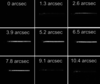Figure 6 Diffraction after two crystals at a distance of 7 m. We rocked the first crystal and registered a strong moving (333) diffraction and a weaker disappearing (444) diffraction.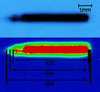Figure 7 X-ray film at a distance of 7 m from the crystal with a (333) diffraction and the (444) and (555) diffractions in background. The original film spot (top) and false colors description (bottom).

The jagged shape of the left-hand side of the diffraction spot in Fig. 6is due to crystal subsurface imperfections. The same imperfection can be seen in Fig. 7.

The horizontal divergence of the bending magnet at BM05 is 2.4 mrad. We observed a 5.16 mm Si(333) diffraction spot at a distance of 7 m after the crystal from a 5 mm impinging beam. This means a divergence of the collimator of only 4.7 arcsec in the horizontal plane, which is much smaller compared with other crystal collimation methods (Ferrari & Korytar, 2001). The horizontal divergence is not coupled to the vertical divergence. The vertical divergence is set by the dispersive setting of the crystals, the cross section of the DuMond diagram, and is independent of the divergence of the impinging beam.

### 4. Conclusions

X-ray collimation as a novel application of diffractive–refractive optics was studied theoretically and experimentally. A set-up with two crystals in a dispersive arrangement successfully demonstrated X-ray collimation in the energy range 6.3–18.8 keV. The experimental results are close to the theoretical calculations of the spectral dependence of the focusing distance. To reach a better agreement between the theory of beam shaping through diffractive–refractive optics and our experiment, further theoretical and experimental research is necessary.

A minor drawback of the device is the higher harmonics contamination, which overlaps on the primary beam. We can overcome this problem by using a special crystal set-up (Hrdý et al., 2011) or by placing a slit between the two crystals. The widths of the diffraction spots in Fig. 5are experimental proof of the collimating optics. An interesting feature of this collimation method is its energy range. Being able to collimate lower energies (below 20 keV) can supplement compact refractive lenses. The degree of collimation is adjustable with a shifting of the value of the focusing distance at which we approximate collimation.

### Acknowledgements

This work was supported by the following funds: Institutional Research Plan AS CR (No. AVOZ 10100522), MPO CR (FR-TI1/412) and MSMT CR (INGO LA 10010 and MSM 0021622410). We would like to thank Mr Lukáš and the company Polovodiče a.s. which provided the crystals.

### ReferencesJOURNAL OFSYNCHROTRONRADIATION